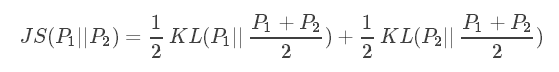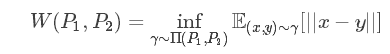# KL散度、JS散度、Wasserstein距离

## 1. KL散度

$KL$$KL$散度又称为相对熵，信息散度，信息增益。$KL$$KL$散度是是两个概率分布P和Q 差别的非对称性的度量。 $KL$$KL$散度是用来 度量使用基于Q的编码来编码来自P的样本平均所需的额外的位元数。 典型情况下，P表示数据的真实分布，Q表示数据的理论分布，模型分布，或P的近似分布。

${D}_{KL}\left(P//Q\right)=-\sum _{x\in X}P\left(x\right)log\frac{1}{P\left(x\right)}+\sum _{x\in X}P\left(x\right)log\frac{1}{Q\left(x\right)}$

1. KL散度不是对称的:$KL\left(A,B\right)$$KL(A, B)$ $\ne$$\neq$ $KL\left(B,A\right)$$KL(B, A)$

## 2. JS散度(Jensen-Shannon)

$JS$$JS$散度度量了两个概率分布的相似度，基于$KL$$KL$散度的变体，解决了KL散度非对称的问题。一般地，$JS$$JS$散度是对称的，其取值是0到1之间。定义如下：$KL$$KL$散度和$JS$$JS$散度度量的时候有一个问题：

## 3. Wasserstein距离

$Wasserstein$$Wasserstein$距离度量两个概率分布之间的距离，定义如下：$\mathrm{\Pi }\left({P}_{1},{P}_{2}\right)$$\Pi (P_1,P_2)$${P}_{1}$$P_1$${P}_{2}$$P_2$分布组合起来的所有可能的联合分布的集合。对于每一个可能的联合分布γ，可以从中采样$\left(x,y\right)\sim \gamma$$(x,y)∼\gamma$得到一个样本x和y，并计算出这对样本的距离||x−y||，所以可以计算该联合分布$\gamma$$\gamma$下，样本对距离的期望值$E\left(x,y\right)\sim \gamma \left[||x-y||\right]$$E(x,y)∼γ[||x−y||]$。在所有可能的联合分布中能够对这个期望值取到的下界$in{f}_{\gamma }$$inf_\gamma$$\mathrm{\Pi }\left({P}_{1},{P}_{2}\right)$$\Pi (P_1,P_2)$E(x,y)$\sim \gamma \left[||x-y||\right]$$∼\gamma[||x−y||]$就是Wasserstein距离。

Wessertein距离相比KL散度和JS散度的优势在于：

KL散度、JS散度、Wasserstein距离